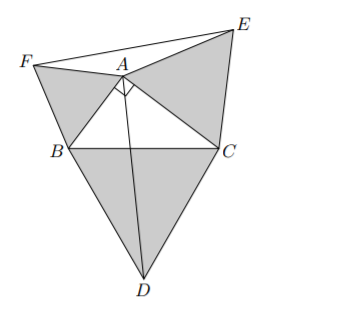# Ratio between weird lengths

Geometry Level 3

In the figure below the triangles BCD, CAE and ABF are equilateral, and the triangle ABC is right-angled.

Determine the fraction $\dfrac{AD}{EF}$ to 3 decimal places×